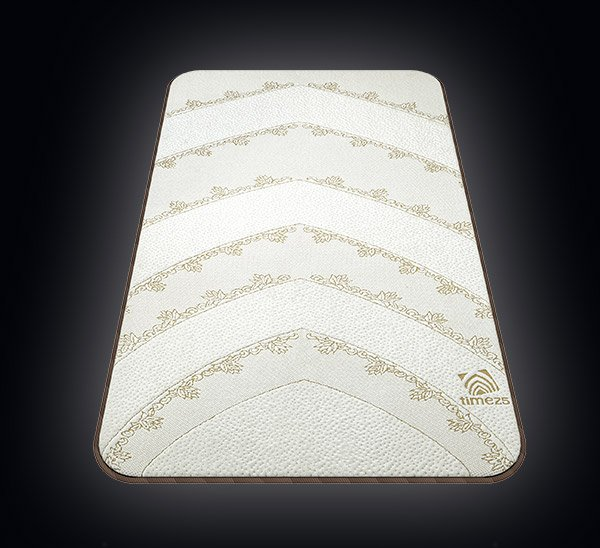• fijr• duhr• asr• magrib• isha| | Mecca, Saudi Arabia

# the science of Muslim Physiologyknee pain

All calculations are based on gross average mass using the average weight of 80KG total gross body weight. Comparisons are based on ergonomic weighted distribution of pressure using body centre of mass calculations against the average weight of comparable items. Comparable timings is based on 30 days for Ramadan and total average prayer practice per day.

52%

of muslims

suffer from
knee pain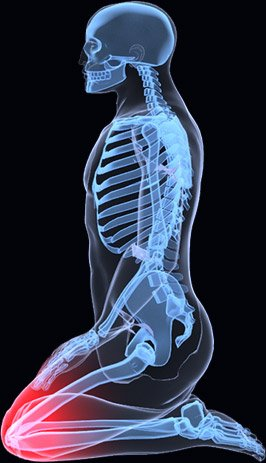back pain

All calculations are based on gross average mass using the average weight of 80KG total gross body weight. Comparisons are based on ergonomic weighted distribution of pressure using body centre of mass calculations against the average weight of comparable items. Comparable timings is based on 30 days for Ramadan and total average prayer practice per day.

20%

of muslims

suffer from
back pain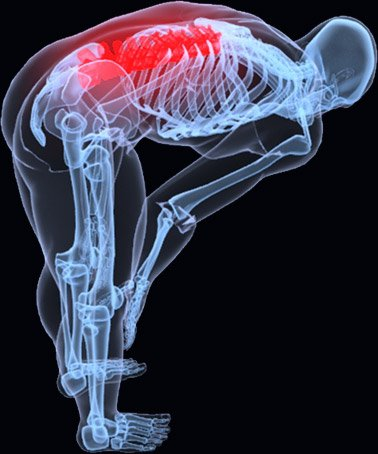neck pain

All calculations are based on gross average mass using the average weight of 80KG total gross body weight. Comparisons are based on ergonomic weighted distribution of pressure using body centre of mass calculations against the average weight of comparable items. Comparable timings is based on 30 days for Ramadan and total average prayer practice per day.

18%

of muslims

suffer from
neck pain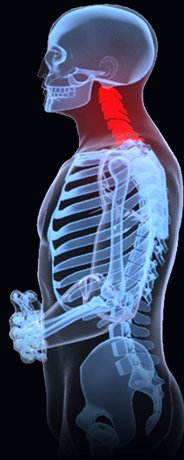inflexibility

All calculations are based on gross average mass using the average weight of 80KG total gross body weight. Comparisons are based on ergonomic weighted distribution of pressure using body centre of mass calculations against the average weight of comparable items. Comparable timings is based on 30 days for Ramadan and total average prayer practice per day.

39%

of muslims

suffer from
inflexibility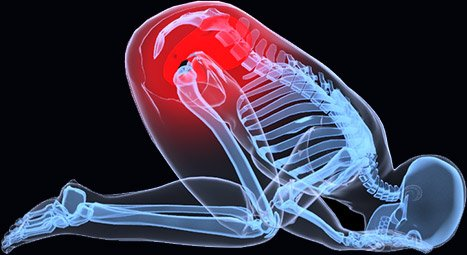posture

All calculations are based on gross average mass using the average weight of 80KG total gross body weight. Comparisons are based on ergonomic weighted distribution of pressure using body centre of mass calculations against the average weight of comparable items. Comparable timings is based on 30 days for Ramadan and total average prayer practice per day.

85%

of muslims

suffer from
posture problems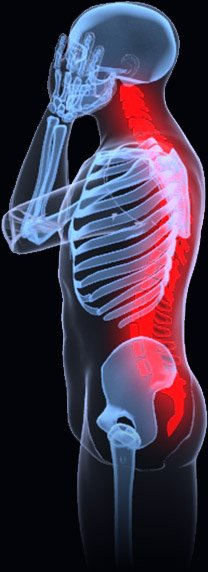the world’s first
physiological prayer mat

pain relief, improved posture support
and a boost of energy